The REG Procedure
 Construction of Q-Q and P-P Plots

If a normal probability-probability or quantile-quantile plot for the variableis requested, thenonmissing values ofare first ordered from smallest to largest: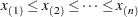If a Q-Q plot is requested (with a PLOT statement of the form PLOT yvariableNQQ.), theth-ordered value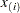is represented by a point with y-coordinateand x-coordinate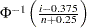, where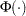is the standard normal distribution.

If a P-P plot is requested (with a PLOT statement of the form PLOT yvariableNPP.), theth-ordered valueis represented by a point with y-coordinateand x-coordinate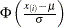, whereis the mean of the nonmissing-values and
is the standard deviation. If an-value has multiplicity(that is,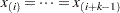), then only the point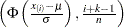is displayed.Previous Page | Next Page | Top of Page